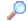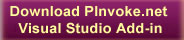SearchDirectory

Desktop Functions:

Smart Device Functions:GlossaryShow Recent ChangesSubscribe (RSS)Misc. Pages
SendInput (user32)

Summary

#### C# Signature:

[DllImport("user32.dll", SetLastError=true)]
static extern uint SendInput(uint nInputs, INPUT [] pInputs, int cbSize);

#### VB.NET Signature:

Private Declare Function SendInput Lib "user32.dll" (ByVal cInputs As Integer, ByRef pInputs As INPUT, ByVal cbSize As Integer) As Integer

#### C# User-Defined Types:

[StructLayout(LayoutKind.Sequential)]
struct MOUSEINPUT
{
int dx;
int dy;
int mouseData;
int dwFlags;
int time;
IntPtr dwExtraInfo;
}

[StructLayout(LayoutKind.Sequential)]
struct KEYBDINPUT
{
short wVk;
short wScan;
int dwFlags;
int time;
IntPtr dwExtraInfo;
}

[StructLayout(LayoutKind.Sequential)]
struct HARDWAREINPUT
{
int uMsg;
short wParamL;
short wParamH;
}

[StructLayout(LayoutKind.Explicit)]
struct INPUT
{
[FieldOffset(0)]
int type;
[FieldOffset(4)]
MOUSEINPUT mi;
[FieldOffset(4)]
KEYBDINPUT ki;
[FieldOffset(4)]
HARDWAREINPUT hi;
}

[DllImport("user32.dll")]

static extern IntPtr GetMessageExtraInfo();

#### VB.NET User-Defined Types:

<StructLayout(LayoutKind.Explicit)> _
Private Structure INPUT
Dim dwType As Integer
Dim mouseInput As mouseInput
Dim keyboardInput As KEYBDINPUT
Dim hardwareInput As hardwareInput
End Structure

<StructLayout(LayoutKind.Explicit)> _
Private Structure KEYBDINPUT
<FieldOffset(0)> Public wVk As Short
<FieldOffset(2)> Public wScan As Short
<FieldOffset(4)> Public dwFlags As Integer
<FieldOffset(8)> Public time As Integer
<FieldOffset(12)> Public dwExtraInfo As IntPtr
End Structure

<StructLayout(LayoutKind.Explicit)> _
Private Structure HARDWAREINPUT
<FieldOffset(0)> Public uMsg As Integer
<FieldOffset(4)> Public wParamL As Short
<FieldOffset(6)> Public wParamH As Short
End Structure

<StructLayout(LayoutKind.Explicit)> _
Private Structure MOUSEINPUT
<FieldOffset(0)> Public dx As Integer
<FieldOffset(4)> Public dy As Integer
<FieldOffset(8)> Public mouseData As Integer
<FieldOffset(12)> Public dwFlags As Integer
<FieldOffset(16)> Public time As Integer
<FieldOffset(20)> Public dwExtraInfo As IntPtr
End Structure

#### Notes:

You'll need 3 constants to use this function,.here they are.

[c#]

const int INPUT_MOUSE = 0;

const int INPUT_KEYBOARD = 1;

const int INPUT_HARDWARE = 2;

[/c#]

I don't know how these definitions would be written in VB.NET, sorry.

*******************

(This code posted by Anh Phuong (http://360.yahoo.com/naphuonghic))

Code VB here:

Dim INPUT_MOUSE As Integer = 0

Dim INPUT_KEYBOARD As Integer = 1

Dim INPUT_HARDWARE As Integer = 2

********************

#### Sample Code:

// It might be better to write this code as a single call to SendInput passing
// all the key downs and keys ups in one array.  If you do this, SendInput
// guarantees that no other keys get inbetween the whole sequence.  As is stands,
// a "real" key transition could in theory sneak in the middle - and if it did
// it would inherit the states of shift, control, etc this program had set up.

class Test
{
public enum VK : ushort
{
SHIFT        = 0x10,
CONTROL          = 0x11,
ESCAPE           = 0x1B,
BACK         = 0x08,
TAB          = 0x09,
RETURN           = 0x0D,
PRIOR        = 0x21,
NEXT         = 0x22,
END          = 0x23,
HOME         = 0x24,
LEFT         = 0x25,
UP           = 0x26,
RIGHT        = 0x27,
DOWN         = 0x28,
SELECT           = 0x29,
PRINT        = 0x2A,
EXECUTE          = 0x2B,
SNAPSHOT         = 0x2C,
INSERT           = 0x2D,
DELETE           = 0x2E,
HELP         = 0x2F,
MULTIPLY         = 0x6A,
SEPARATOR        = 0x6C,
SUBTRACT         = 0x6D,
DECIMAL          = 0x6E,
DIVIDE           = 0x6F,
F1           = 0x70,
F2           = 0x71,
F3           = 0x72,
F4           = 0x73,
F5           = 0x74,
F6           = 0x75,
F7           = 0x76,
F8           = 0x77,
F9           = 0x78,
F10          = 0x79,
F11          = 0x7A,
F12          = 0x7B,
OEM_1        = 0xBA,   // ',:' for US
OEM_PLUS         = 0xBB,   // '+' any country
OEM_COMMA        = 0xBC,   // ',' any country
OEM_MINUS        = 0xBD,   // '-' any country
OEM_PERIOD       = 0xBE,   // '.' any country
OEM_2        = 0xBF,   // '/?' for US
OEM_3        = 0xC0,   // '`~' for US
MEDIA_NEXT_TRACK     = 0xB0,
MEDIA_PREV_TRACK     = 0xB1,
MEDIA_STOP           = 0xB2,
MEDIA_PLAY_PAUSE     = 0xB3,
LWIN       =0x5B,
RWIN       =0x5C
}

public struct KEYBDINPUT
{
public ushort wVk;
public ushort wScan;
public uint dwFlags;
public long time;
public uint dwExtraInfo;
};
[StructLayout(LayoutKind.Explicit,Size=28)]
public struct INPUT
{
public uint type;
public KEYBDINPUT ki;
};

void Test()
{
INPUT structInput;
structInput = new INPUT();
structInput.type = Win32Consts.INPUT_KEYBOARD;

// Key down shift, ctrl, and/or alt
structInput.ki.wScan = 0;
structInput.ki.time = 0;
structInput.ki.dwFlags = 0;
structInput.ki.dwExtraInfo = Win32.GetMessageExtraInfo();
if (Shift)
{
structInput.ki.wVk = (ushort)VK.SHIFT;
intReturn = Win32.SendInput(1, ref structInput, (UInt32)sizeof(INPUT));
}
if (Ctrl)
{
structInput.ki.wVk = (ushort)VK.CONTROL;
intReturn = Win32.SendInput(1, ref structInput, (UInt32)sizeof(INPUT));
}
if (Alt)
{
intReturn = Win32.SendInput(1, ref structInput, (UInt32)sizeof(INPUT));
}
if (Win)
{
structInput.ki.wVk = (ushort)VK.LWIN;
intReturn = Win32.SendInput(1, ref structInput, (UInt32)sizeof(INPUT));
}
// Key down the actual key-code
structInput.ki.wVk = vk;
intReturn = Win32.SendInput(1, ref structInput, (UInt32)sizeof(INPUT));
// Key up the actual key-code
structInput.ki.dwFlags = Win32Consts.KEYEVENTF_KEYUP;
intReturn = Win32.SendInput(1, ref structInput, (UInt32)sizeof(INPUT));
// Key up shift, ctrl, and/or alt
if (Shift)
{
structInput.ki.wVk = (ushort)VK.SHIFT;
intReturn = Win32.SendInput(1, ref structInput, (UInt32)sizeof(INPUT));
}
if (Ctrl)
{
structInput.ki.wVk = (ushort)VK.CONTROL;
intReturn = Win32.SendInput(1, ref structInput, (UInt32)sizeof(INPUT));
}
if (Alt)
{
intReturn = Win32.SendInput(1, ref structInput, (UInt32)sizeof(INPUT));
}
if (Win)
{
structInput.ki.wVk = (ushort)VK.LWIN;
intReturn = Win32.SendInput(1, ref structInput, (UInt32)sizeof(INPUT));
}
}
}

#### VB.NET Sample Code:

Imports System.Runtime.InteropServices

Public Class Form1

Private tb As New TextBox
Private WithEvents but1 As New Button

Sub New()
InitializeComponent()
tb = New TextBox : tb.Location = New Point(10, 10)
but1 = New Button : but1.Location = New Point(10, 40)
but1.Text = "example"
End Sub

Private Sub DoMouse(ByVal flags As NativeMethods.MOUSEEVENTF, ByVal newPoint As Point)
Dim input As New NativeMethods.INPUT
Dim mi As New NativeMethods.MOUSEINPUT
input.dwType = NativeMethods.InputType.Mouse
input.mi = mi
input.mi.dwExtraInfo = IntPtr.Zero
' mouse co-ords: top left is (0,0), bottom right is (65535, 65535)
' convert screen co-ord to mouse co-ords...
input.mi.dx = newPoint.X * (65535 / Screen.PrimaryScreen.Bounds.Width)
input.mi.dy = newPoint.Y * (65535 / Screen.PrimaryScreen.Bounds.Height)
input.mi.time = 0
input.mi.mouseData = 0  ' can be used for WHEEL event see msdn
input.mi.dwFlags = flags
Dim cbSize As Integer = Marshal.SizeOf(GetType(NativeMethods.INPUT))
Dim result As Integer = NativeMethods.SendInput(1, input, cbSize)
If result = 0 Then Debug.WriteLine(Marshal.GetLastWin32Error)
End Sub

Private Sub DoKeyBoard(ByVal flags As NativeMethods.KEYEVENTF, ByVal key As Keys)
Dim input As New NativeMethods.INPUT
Dim ki As New NativeMethods.KEYBDINPUT
input.dwType = NativeMethods.InputType.Keyboard
input.ki = ki
input.ki.wVk = Convert.ToInt16(key)
input.ki.wScan = 0
input.ki.time = 0
input.ki.dwFlags = flags
input.ki.dwExtraInfo = IntPtr.Zero
Dim cbSize As Integer = Marshal.SizeOf(GetType(NativeMethods.INPUT))
Dim result As Integer = NativeMethods.SendInput(1, input, cbSize)
If result = 0 Then Debug.WriteLine(Marshal.GetLastWin32Error)
End Sub

Private Sub but1_Click(ByVal sender As Object, ByVal e As System.EventArgs) Handles but1.Click
'move to the textbox, click it to focus, keydown some chars...
' get textbox location in screen co-ords
Dim tbLocation As Point = Me.PointToScreen(Me.tb.Location)
' nudge it into the tb
tbLocation.X += 5
tbLocation.Y += 5
' move to the TB. it is a MOVE event, and we use ABSOLUTE co-ordinates
DoMouse(NativeMethods.MOUSEEVENTF.MOVE Or NativeMethods.MOUSEEVENTF.ABSOLUTE, tbLocation)
' click the TB
DoMouse(NativeMethods.MOUSEEVENTF.LEFTDOWN, New Point(0, 0))
' release the mouse
DoMouse(NativeMethods.MOUSEEVENTF.LEFTUP, New Point(0, 0))
' the key codes are virtual keycodes (see mdsn)
' I'm using a shortcut to save space - casting chars to ints
' if you want to change case, you need to send a shift key with the letter...
Dim message As String = "HELLO WORLD"
For Each c As Char In message
Dim key As UInteger = Convert.ToInt16(c)
DoKeyBoard(0, key)  ' key down is 0 - no flag...
DoKeyBoard(NativeMethods.KEYEVENTF.KEYUP, key)
Next
DoKeyBoard(0, Keys.Decimal) ' example using keys enum which I think matches the VKEY values
DoKeyBoard(NativeMethods.KEYEVENTF.KEYUP, Keys.Decimal)
End Sub

End Class

Public Class NativeMethods

<DllImport("user32.dll", SetLastError:=True)> _
Friend Shared Function SendInput(ByVal cInputs As Int32, ByRef pInputs As INPUT, ByVal cbSize As Int32) As Int32
End Function

<StructLayout(LayoutKind.Explicit, pack:=1, Size:=28)> _
Friend Structure INPUT
<FieldOffset(0)> Public dwType As InputType
<FieldOffset(4)> Public mi As MOUSEINPUT
<FieldOffset(4)> Public ki As KEYBDINPUT
<FieldOffset(4)> Public hi As HARDWAREINPUT
End Structure

<StructLayout(LayoutKind.Sequential, pack:=1)> _
Friend Structure MOUSEINPUT
Public dx As Int32
Public dy As Int32
Public mouseData As Int32
Public dwFlags As MOUSEEVENTF
Public time As Int32
Public dwExtraInfo As IntPtr
End Structure

<StructLayout(LayoutKind.Sequential, pack:=1)> _
Friend Structure KEYBDINPUT
Public wVk As Int16
Public wScan As Int16
Public dwFlags As KEYEVENTF
Public time As Int32
Public dwExtraInfo As IntPtr
End Structure

<StructLayout(LayoutKind.Sequential, pack:=1)> _
Friend Structure HARDWAREINPUT
Public uMsg As Int32
Public wParamL As Int16
Public wParamH As Int16
End Structure

Friend Enum InputType As Integer
Mouse = 0
Keyboard = 1
Hardware = 2
End Enum

<Flags()> _
Friend Enum MOUSEEVENTF As Integer
MOVE = &H1
LEFTDOWN = &H2
LEFTUP = &H4
RIGHTDOWN = &H8
RIGHTUP = &H10
MIDDLEDOWN = &H20
MIDDLEUP = &H40
XDOWN = &H80
XUP = &H100
VIRTUALDESK = &H400
WHEEL = &H800
ABSOLUTE = &H8000
End Enum

<Flags()> _
Friend Enum KEYEVENTF As Integer
EXTENDEDKEY = 1
KEYUP = 2
UNICODE = 4
SCANCODE = 8
End Enum

End Class

#### Alternative Managed API:

Here is a managed solution: I think it works only in 2.0. Someone please verify.

http://msdn.microsoft.com/library/default.asp?url=/library/en-us/cpref/html/frlrfSystemWindowsFormsSendKeysClassTopic.asp

Please note that there is an unresolved issue using the SendKeys class in Windows Vista RC1 (Build 5536).

More specifically you may encounter a "Hook cannot be created." exception.

Note

Do you know one? Please contribute it!

Documentation
SendInput on MSDN

Do you have...

• helpful tips or sample code to share for using this API in managed code?
• corrections to the existing content?
• variations of the signature you want to share?
• additional languages you want to include?

Select "Edit This Page" on the right hand toolbar and edit it! Or add new pages containing supporting types needed for this API (structures, delegates, and more).Access PInvoke.net directly from VS:Terms of UseFind ReferencesShow Printable VersionRevisions Show changes